# 5 Modeling Calculations

• Create formulas.
• Setting up a calculation model.
• Copy formulas and absolute and relative cell addresses.
• Formulas that evaluate to TRUE or FALSE.
• Use of cell names.

One of the most important aspects of working with Excel is to perform calculations. You need formulas in these calculations. To work effectively and efficiently with formulas, you need to think in advance carefully about what to put where in the worksheet. This can save you a lot of time and trouble.

Calculations are performed using formulas. Formulas contain numbers or references to cells with numbers. Between the numbers, there are the symbols for the calculations to be carried out, such as +, -, *,/, etc.

A formula should always begin with the = character. It is possible to start a formula with a +, but in that case, Excel translates this in = after entering the data.

To enter a formula you start typing = and then you enter the calculation as you would do it on a calculator. Instead of numbers, you also can type cell addresses or cell names where the numbers are. Also, instead of typing in the cell addresses, you can designate it with the mouse.

In the formulas you can use the following symbols (operators) for calculations:

Table 5.1: Operators.
symbol meaning example result
+ addition =4+5 9
- subtraction =29-6 23
* multiplication =7*8 56
/ division =6/2 3
^ exponentiation =2^3 8
() parentheses for operator precedence =30-(4+6) 20

Practice with these examples in a worksheet.

This is an exercise for a very simple calculation, see figure 5.1. Three cells contain numbers:

• C1, the purchase amount.
• B2, the profit rate.
• B5, the VAT rate.

Four cells (C2, C3, C5, and C6) contain formulas. The idea is that if you change one or more of the values in C1, B2 or B5 all the depending values are automatically adjusted.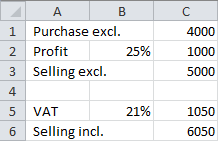Figure 5.1: Example calculation purchase, profit and selling.

2. Type the texts you see in column A. Some texts don’t fit within the column width. That is not important at the moment. If desired, you can make the column wider.

3. In cell B2 enter the value 25%, in cell B5 the value 21% and in cell C1 the value 4000.

4. Enter in cell C2 the formula =B2*C1. After pressing the Enter key, the result of the formula, 1000, is displayedin cell C2. This is the calculated profit.

5. Enter in cell C3} the formula =C1+C2. Upon completion of the input in cell C3 the result, 5000, is displayed.

6. Enter in cell C5 the formula =B5*C3. After completion in cell C5 the result, 1050, is displayed.

7. Enter in cell C6 the formula =C3+C5. After completion in cell C6 the result, 6050, is displayed..

8. Experiment with other values for purchase, profit rate, and VAT rate.

Note that by changing the values, all formulas will be automatically recalculated.

## 5.2 Task: Calculate working hours

In figure 5.2, you see a worksheet where formulas are used to calculate the wages by multiplying the hours with an hourly rate. The total is obtained by addition.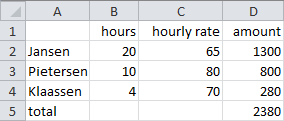Figure 5.2: Example calculation hours, hour rate and totals.

File: Wages.xlsx

1. Open the practice file.

2. Enter in the column amount the correct formulas:

• in cell D2 the formula =B2*C2
• in cell D3 the formula =B3*C3
• in cell D4 the formula =B4*C4
• in cell D5 the formula =D2+D3+D4

## 5.3 A calculation model

Using Excel, you can make all kinds of calculations. For a larger and more complex calculation, careful thinking about how setting up the calculation in a worksheet is needed.

In a calculation model, you always have to deal with three types of cells.

Input
In these cells, the user continuously enters different values. In fact, these are the cells for the variables.
Calculations
In these cells, all kinds of calculations with the values from the input cells are carried out.
Output
In these cells, the formatted results are displayed.

The data flow in the model is from the input through the calculation to the output. When setting up a model you should think in the opposite direction. After all, the desired result is the point. From the desired results the necessary calculations are derived. And these calculations determine what input is necessary.

There are some guidelines for entering a model in Excel:

1. Make a separation between input, calculation, and output. For smaller models, this can be in separate places in the worksheet. For example, input at the top of the worksheet. For large models, it is better to use separate sheets.Figure 5.3: Design of a calculation model.At the left 1 worksheet, at the right 3 worksheets.

1. Ensure that a worksheet can be read like a book, from top to bottom and from left to right.

2. Find out what numbers can change, for example, the price of an item. Put these numbers in individual cells. These numbers are variables.

3. Use as little numbers as possible in the formula itself, but use references to cells where the numbers are. Do the same for numbers that hardly change over time, such as the VAT rate.

4. Always make the purpose of the cell content visible by putting an explanatory text in a neighboring cell (before, above, …).

5. Put headers above columns with numbers and before rows with numbers.

6. If possible, make one kind of formula per row or per column. The advantage of this is that the formulas can be copied easily across rows or columns.

7. Divide a complicated formula into multiple pieces so that intermediate results (e.g. subtotals ) can be checked. This prevents errors in formulas.

8. When using multiple worksheets for the model give these sheets obvious names.

9. Take care of some documentation, especially for larger models, and put this in separate worksheets.

## 5.4 Copying formulas

It is very useful to be able to copy formulas to other cells, especially when formulas are very similar to each other. In the following figure, you see an example where the formulas in A3, B3, C3, and D3 show great similarities. This makes it possible to enter the formula only in cell A3 and then copy it to B3:D3.

A (cell)range is a group of cells that you can refer to in a formula. This range is usually rectangular, starting with the top left cell, then a colon, then the bottom right cell. Examples: B2:E7, B3:E3, D2:D6.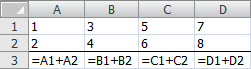Figure 5.4: Example with copyable formulas.

Because a formula often contains cell references it is important to consider what to do with these cell references during the copy action. There are two possibilities:

• The cell references adjust to a new situation. The cell addresses in the formula are relative.
• The cell references don’t change and remain the same. The cell addresses in the formula are absolute.

An address is made absolute by putting dollar signs in the address. You can place a dollar sign for the column letter and/or for the row number. The part that contains the dollar sign is absolute and will not be changed during copying. There are four possibilities for a cell address:

Type Example
Relative address B1
Absolute column and absolute row address $B$1
Absolute column and relative row address $B1 Relative row and absolute column address B$1

When copying a formula with cell addresses, the absolute part is not modified and the relative part will be modified.

You can change the type of a cell reference in one of the following ways:

• Type the dollar signs at the desired place.
• If the cursor is in a cell address, press repeatedly on key F4, until the desired result is achieved. During repetitive pressing on F4 all four possibilities will be displayed.

### Copy rules

If you copy formulas with cell references Excel applies the following rules:

• By copying to the left or to the right direction the column letter will be lowered or raised.
• By copying in the direction upwards or downwards the row number will be decreased or increased.
Formula Copy action Result
=A1+B2 1 cell to the right =B1+C2
1 cell down =A2+B3
1 cell to the right and 1 cell down =B2+C3
=A$1+B$2 1 cell to the right =B$1+C$2
1 cell down =A$1+B$2
1 cell to the right and 1 cell down =B$1+C$2
=$A1+$B2 1 cell to the right =$A1+$B2
1 cell down =$A2+$B3
1 cell to the right and 1 cell down =$A2+$B3
=$A$1+$B$2 each copy action =$A$1+$B$2

In figure 5.5, you see a list of insurance premiums for cars. The list starts at a value of $10.000 and a premium of$ 675. The next values grow with increments of $1000 and the premium with increments of$ 45. Make this list using formulas for value and premium in the rows 6 until 20.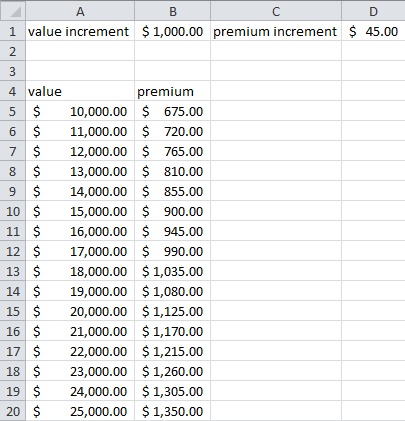1. Open the practice file.

2. Format the values as currencies with the button on the ribbon (Home > button Accounting Number Format (group Number)]{.uicontrol}. Adjust also the column widths so that all contents fit.

3. Enter in cell A6 the formula =A5+$B$1.

4. Enter in cell B6 the formula =B5+$D$1.

5. Select the area A6:B6 and drag the fill handle until B20.

## 5.5 True/False formulas

Some formulas don’t perform calculations, but rather compare two things. Such formulas have only two possible outcomes: TRUE or FALSE.

In these formulas you can use the following operators:

Table 5.2: Operators for comparing two items.
Operator Meaning
= equal
<> not equal to
< less than
<= less than or equal to
> greater than
>= greater than or equal to

In table 5.3 are some examples of such formulas. To understand its functioning well, you could enter these in a cell. The parentheses you see in the formulas are not strictly necessary, but provide more clarity on the intent of the formula. Therefore it is recommended to always use parentheses.

Table 5.3: Examples of TRUE/FALSE formulas.
Formula Result
=(1=1) TRUE
=(1=2) FALSE
=(1<>1) FALSE
=(1<>2) TRUE
=(1<1) FALSE
=(1<2) TRUE
=(1<=1) TRUE
=(1<=2) TRUE
=(1>2) FALSE
=("a"="b") FALSE
=("a"<>"b") TRUE

In operations, text should always be placed between double-quotes.

Just putting such formulas in cells does not make much sense. However, they can appear in calculations. In that case Excel uses the following numerical values:

• TRUE = 1
• FALSE = 0

Because of these numerical values you can calculate with it and create conditions in a calculation. The result depends of the outcome of the condition. In the following example, the postage for orders depends on the ordered quantity.

At an internet shop, an order is delivered without postage for orders from $25. In the next figure, you can see that the postage is calculated with a true/false formula. At the beginning of the formula is (B5<$B$2). This part has two possible outcomes, TRUE (=1) or FALSE (=0). And this result is again multiplied by$3,50. There are only two possible outcomes:

• 1 * 3.50 = 3.50
• 0 * 3.50 = 0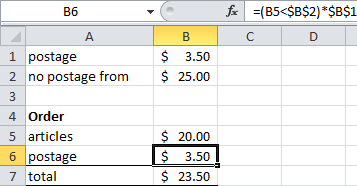Figure 5.6: Example with a true/false formula.

Create this example and check the correct working by entering different values in the cells B1, B2, and B5.

## 5.6 Cell Names

The use of meaningful names for cells and the rules for naming.

Working with cell addresses in formulas can be confusing, formulas are hard to read and it is difficult to see what the intention of the formula is. Fortunately, you can give meaningful names to cells and areas. A formula for example can then be =Sales-Purchases.

Rules for naming:

• Names should begin with a letter or with the underscore symbol (-), digits are allowed after that. It is not allowed to start a name with a digit.

• Names cannot contain spaces. To simulate a space you can use the underscore symbol, for example, [Sales_2010](.varname}.

• Most symbols (comma, colon, exclamation point, etc.) can not be used.

In this task, you will learn a few ways to assign names to cells and use them in formulas.

There are several ways to assign a name to a cell. The method used in practice usually depends on the preferences of the user and their entering speed. In this task, there are two ways proposed to assign a name to a cell and three methods to use the name in a formula. The same methods can be used to assign a name to an area of cells.

2. Enter in A1 the number 100 and in A2 the number 275.

3. Select A1 and choose Formulas > Define Name (group Defined Names).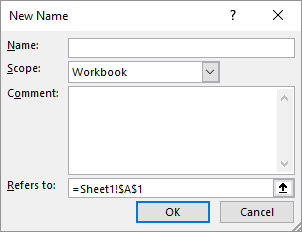Figure 5.7: Dialog box New Name.

In the Name text box you can enter the name. Sometimes Excel suggests a name. In the Comment box, you can optionally give an explanation, which can be useful later (audits) for checking and creating documentation for the model.

1. Enter the name Purchases and click on OK. The name box now displays this name instead of the address.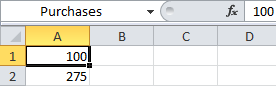Figure 5.8: Cell A1, now with the name Purchases in the name box instead of the address.

An alternative and quick way to assign a name is by selecting a cell and then directly enter the name in the name box. This is done for cell A2 in the next step.

1. Select cell A2. Click in the Name box and change A2 in Sales and press Enter.

2. Select cell A3 and enter the formula for calculating the profit in one of the following ways:

• Type = Sales-Purchases and press the Enter key.
• Type = then select A2, type -, then [select A1 and press the Enter key.
• Type = then choose Formulas > Use in formula (group Defined Names) > Sales, type - and then choose Formulas > Use in formula (group Defined Names) > Purchases and press the Enter key.

In cell A3 the answer 175 appears. Selecting this cell shows you =Sales-Purchases in the formula bar. By using names you get a well readable formula.

### Task: Using names in existing formulas

When you assign a name to a cell or cell range, Excel automatically uses them in new formulas, but not in already existing formulas. In the next example, cell B5 contains the formula =B2+B3+B4. When you assign names to the cells B2, B3, and B4, the formula remains unchanged.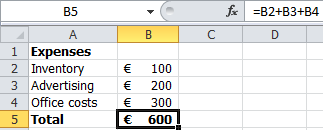However, you can quite easily use new names in existing formulas. In the practice file of this example, the cells in the formula already have names. Through the following steps, the addresses in the formula will be replaced by their names.

File: Office-Expenses.xlsx

1. Open the practice file.

2. Select cell B5.

3. Choose Formulas and click on the arrow from the button Define Name (group Defined Names) and choose Apply Names.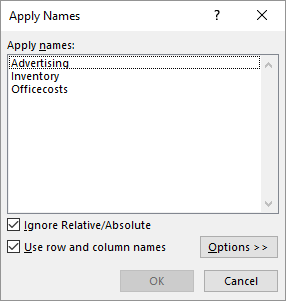Figure 5.10: Dialog box apply names.

1. Select all names and click OK. The formula in cell B5 now is =Inventory+Advertising+Officecosts.

To select multiple names, hold down the Ctrl key.

Excel has capabilities for managing names. You can get a list of all names in the workbook, and you can change and remove names. Especially for the larger workbooks containing many names, this is an indispensable means for the management of names.

File: Expenses.xlsx

1. Open the practice file.

2. Choose Formulas > Name Manager (group Defined Names).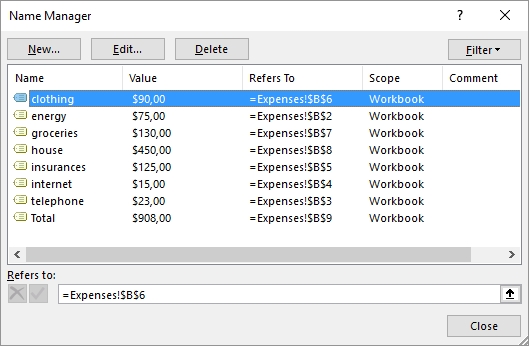Figure 5.11: Name manager.

Through this dialog box you can perform various operations with the names.

• Deleting name: Select the name and choose Delete.

• Changing name: Select the name and choose Edit.

• Comment creating/editing: Select the name and choose Edit.

• Create a new name: Select the name and choose New.

How to create a list of all existing names and corresponding addresses.

Documenting a spreadsheet also includes a list of all names in use and the corresponding cells. Excel can make such a list for you and put it on a worksheet, but the way to do that is not so obvious. It is recommended to create such a list of names in a new worksheet.

File: Expenses.xlsx

1. Open the practice file.

2. Create a new sheet and select cell A1.

3. Choose Formulas > Use in formula (group Defined Names) > Paste Names > Paste List.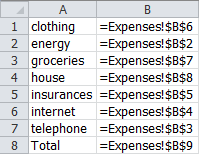Figure 5.12: List with cell names and addresses.

## 5.7 Case: Price calculation articles

Creating a model, using formulas and copying formulas.

A shop at the international airport sells articles both tax-free and with 21% VAT. The currency for tax-free prices is the U.S. dollar, but customers can also pay in euros. For the VAT and the prices with VAT only the euro will be used. In figure 5.13, you can see a part of the price table. Article numbers and dollar prices are somewhat artificial to practice several Excel skills. The intention is to create this table as convenient as possible. Only some indications are given, no detailed steps.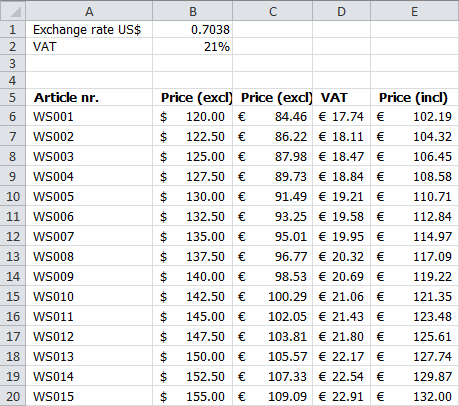Figure 5.13: Price table

1. The dollar exchange rate and the VAT percentage are input variables for the calculation and should be placed at the top of the worksheet, separated from the price table. An explanatory text is in the cells before.

2. A pattern is in the article numbers. You only need to type in the first two numbers, select these numbers and then drag down.

3. The dollar prices have also a pattern (increments of 2.50). Enter only the first two prices, select them and drag down. The prices themselves must be formatted as currency with 2 decimals and the \$ as currency symbol.

4. The price in euro can be calculated from the dollar price by multiplying this price with the dollar exchange rate. You only need to enter the correct formula in the first cell (C6) then you can copy it down. Use an absolute address for the dollar exchange rate, this address should not be updated during the copy action.

5. To calculate the amount of VAT the euro price should be multiplied by the VAT rate. And for the price incl. VAT the amount of VAT must be added to the exclusive price. Again, you just have to enter the correct formulas in the first cells (D6 and E6) and then copy it down. Use an absolute address for the VAT rate.

6. Finally, all euro amounts should be formatted as currencies.